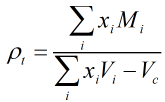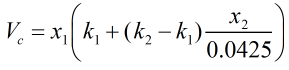# C183 – ISO 6578 Klosek-McKinley LNG Density

## Description

This equation is used to calculate the saturated liquid density of LNG mixtures from composition. The equation is valid at temperatures between -180°C and -140°C, and only for LNG mixtures with:

• an average molecular mass of less than 20.0 kg/kmol,
• mole fractions of N2 less than 5%,
• mole fractions of total C4 less than 5%,
• mole fractions of C5+ less than 1%,
• and no more than a “trace” of O2.

ISO 6578:1991 (BS 7577:1992) – Calculation procedures for static measurement of refrigerated light hydrocarbon fluids

KELTON calculation reference C183

FLOCALC calculation reference F054

KIMS calculation references K187

## Inputs

The required inputs for this calculation are:

• LNG mixture composition
• Temperature at which the saturated density is required

## Calculations

The LNG density is calculated from the following equation:Where ρt = LNG mixture density at temperature t in °C xi = Mole fraction of component i Mi = Molar mass of component i Vi = Molar volume of component i at temperature t in °C Vc = Reduction in volume due to mixing of components at temperature t in °C

The molar mass and volume values are obtained from the relevant tables in ISO 6578:1991.

The reduction in volume due to mixing, Vc, is calculated from:Where k1 = Correction factor due to presence of hydrocarbons k2 = Correction factor due to presence of nitrogen x1 = Mole fraction of methane x2 = Mole fraction of nitrogen

The correction factors k1 and k2 are obtained from the relevant tables in ISO 6578:1991, as a function of temperature and average molecular weight of the LNG mixture.

Back